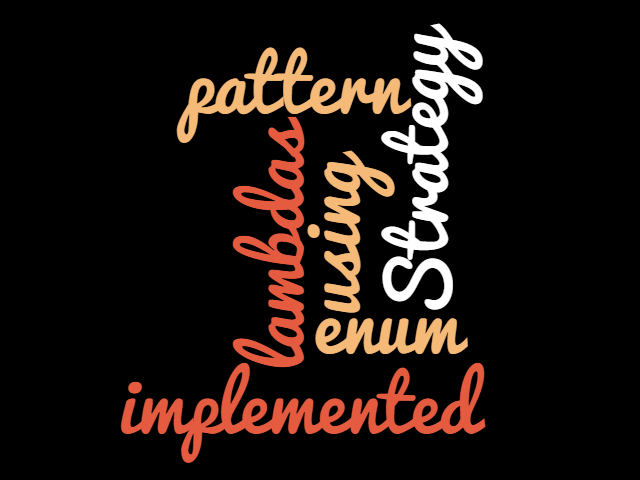News Ticker

# Enum Strategy Pattern with LambdasIn this post I would like to show how the strategy pattern can be implemented as an enum with lambdas.

The Strategy Pattern is one of the Gang of Four design patterns published in thier book: Elements of Reusable Object book. The intent of the strategy pattern is:

Define a family of algorithms, encapsulate each one, and make them interchangeable. Strategy lets the algorithm vary independently from clients that use it.

We will develop a simple calculator where the strategies are basic arithmetic operations.

We start with an interface that defines a single abstract method.

[sourcecode language=”java” toolbar=”false”]

@FunctionalInterface
public interface OperationStrategy {
T compute(T x, T y);
}

[/sourcecode]

We now implement each arithmetic operation using a lambda expression.

[sourcecode language=”java” toolbar=”false”]
public enum Operation implements OperationStrategy {

ADD((x, y) -&gt; x + y),
SUBTRACT((x, y) -&gt; x – y),
MULTIPLY((x, y) -&gt; x * y),
DIVIDE((x, y) -&gt; x / y),
MAX(Double::max);

private OperationStrategy operationStrategy;

Operation(final OperationStrategy operationStrategy) {
this.operationStrategy = operationStrategy;
}

@Override
public Double compute(Double x, Double y) {
return operationStrategy.compute(x, y);
}

}
[/sourcecode]

A series of tests prove that it works.

[sourcecode language=”java” toolbar=”false”]
@RunWith(MockitoJUnitRunner.class)
public class OperationStrategyTest {

@Test
public void shouldAddTwoNumbers() {
assertThat(Operation.ADD.compute(5d, 5d))
.isEqualTo(new Double(10));
}

@Test
public void shouldSubtractTwoNumbers() {
assertThat(Operation.SUBTRACT.compute(10d, 5d))
.isEqualTo(new Double(5d));
}

@Test
public void shouldMultiplyTwoNumbers() {
assertThat(Operation.MULTIPLY.compute(5d, 5d))
.isEqualTo(new Double(25));
}

@Test
public void shouldDivideTwoNumbers() {
assertThat(Operation.DIVIDE.compute(10d, 2d))
.isEqualTo(new Double(5d));
}

@Test
public void shouldDetermineMaximumOfTwoNumbers() {
assertThat(Operation.MAX.compute(10d, 5d))
.isEqualTo(new Double(10d));
}
}
[/sourcecode]

The use of lambdas as strategies reduces boilerplae code quite substantially, though it would not be correct to lambdas if the strategy is complex and requires a lot of code. It would become cumbersome.

A git repository of this code is available here: https://github.com/atheedom/Java8

Other articles that you might find interesting: